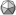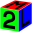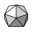Home / Section index www.icosaedro.it#M2 - Examples gallery

These little programs illustrate some of the features of the M2 programming language. The example are ordered from simpler to more complexes. Select an example and try it on-line. You may see the source code and you may chage it. The output of the programs is presented on a page of plain text. Some programs try to simulate graphics using characters: not very exciting, but sufficient to the purpuse of the example.00-HelloWorld.mod The basic of all the programs from any time ever: hello world!10-PythagorasTables.mod Print the multiplication tables. An example of nested FOR loops.15-ReverseString.mod Reverse the characters in a string using a recursive method. Demostrate the usage of the substrings and of the recursive functions.20-ComplexNumbers.mod Compute the parallel between a resistance, a capacitor and an inductance. Illustrate a basic library for complex numbers calculations and the usage of the RECORDs.25-Primes.mod This program computes a list of prime numbers. It illustrate the usage of arrays, loops and control structures. From: Programming in Oberon, N.Wirth, ch. 1.30-FunctionPlot.mod Plot of the graphic of a function (faded oscillator). A virtual "graphical screen" is simulated with a matrix of charactes and some primitive drawing functions to plot points and stright segments are given.31-FunctionPlot.mod Plot of the graphic of a function (faded oscillator). Uses the module "img" to create a GIF at high resolution.40-RotatingCube.mod Wireframe representation of a rotating cube. We use the library "m3d" to do rotations and translations. The graphical screen is simulated with a matrix of characters.41-RotatingCube.mod Wireframe representation of a rotating cube. We use the library "m3d" to do rotations and translations. We use the module "img" to create a GIF image.50-BinaryTree.mod Classical binary tree: insertion, deletion, traversing. Illustrate handling of dynamical data structures and function recursion.60-Cipher.mod Here we encrypt a secret messages using 3DES with a 192 bit key. This illustrates the library for symmetric crittography.70-ExprParser.mod Simple expression parser. The syntax of the expression is as follow: expression = ["-"] termin { ("+"|"-") termin }; termin = factor { ("*"|"/") factor }; factor = letter | "(" expression ")"; The expression is parsed and the program generate the instructions of a virtual stack machine needed to compute the result.
```
```
 Umberto Salsi Comments Contact Site map Home / Section index
Still no comments to this page. Use the Comments link above to add your contribute.